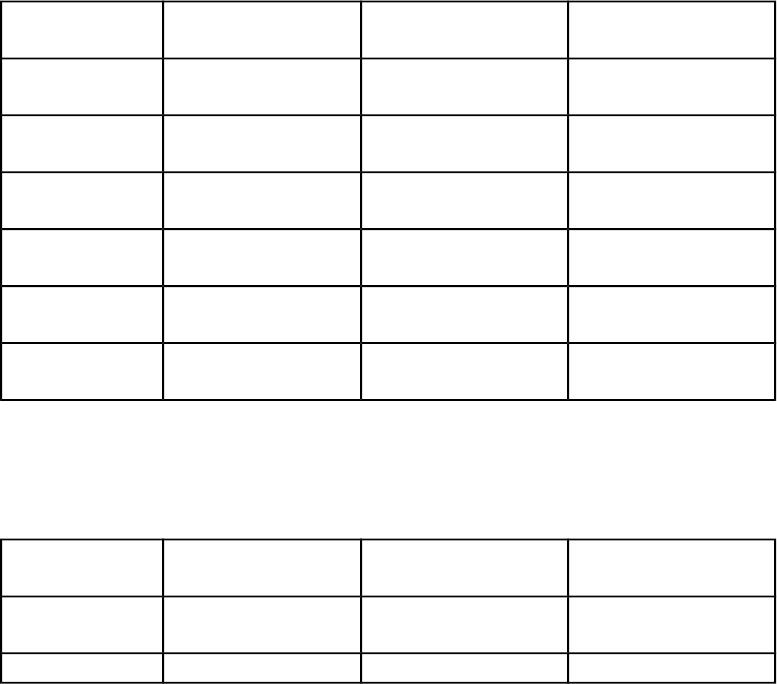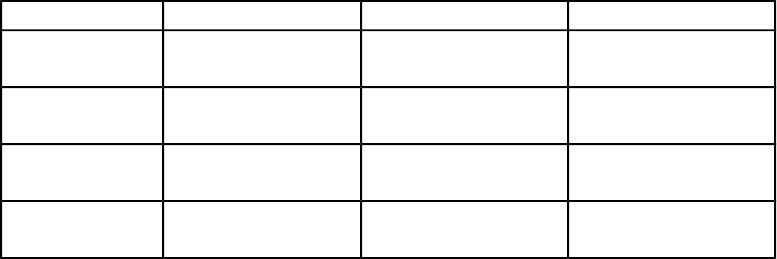Type
Quiz
Book Title
Basic Marketing Research: Using Microsoft Excel Data Analysis 3rd Edition
ISBN 13
978-0135078228

### 978-0135078228 Chapter 12 Lecture Note

June 7, 2019
CHAPTER 12
LEARNING OBJECTIVES
To find out what it means to generalize the findings of a survey
To understand that a sample finding is used to estimate a population fact
To discover how to estimate a confidence interval for a percentage or an average
To learn how to test a hypothesis about a population percentage or an average
To become familiar with the “Generalize” functions of the XL Data Analyst
CHAPTER OUTLINE
Generalizing a Sample’s Findings
Estimating the Population Value
How to Estimate a Population Percentage (Categorical Data)
Calculating a Conference Interval for a Percentage
Interpreting a 95% Confidence Interval for a Percentage
How to Obtain a 95% Confidence Interval for a Percentage with XL Data Analyst
How to Estimate a Population Average (Metric Data)
Calculating a Confidence Interval for an Average
Interpreting a Confidence Interval for an Average
How to Obtain a 95% Confidence Interval for an Average with XL Data Analyst
1
Flow Chart of Generalization Analysis for Confidence Intervals
Testing Hypotheses About Percents of Averages
Testing a Hypothesis About a Percentage
Why Use the 95% Significance Level?
How Do We Know That We Have Made the Correct Decision?
Testing a Directional Hypothesis
How to Test a Hypothesis About a Percentage with XL Data Analyst
Is It t or z? And Why You Do Not Need to Worry About It
Testing a Hypothesis About an Average
How to Test a Hypothesis About an Average with XL Data Analyst
Flow Chart of Generalization Analysis for Hypothesis Tests
How to Present Generalization Analyses
Guidelines for Confidence Intervals
The General Case
The Findings-Specific Case
Guidelines for Hypothesis Tests
KEY TERMS
Confidence interval
Directional hypothesis
Generalization
Parameter
“Parameter estimation”
Population fact
2Hypothesis
Hypothesis testing
Intuitive hypothesis testing
Most commonly used level of confidence
Null hypothesis
Sample finding
Sampling distribution
Standard error
Standard error of a percentage
Standard error of the average
TEACHING SUGGESTIONS
1. The effect of sample size on a confidence interval can be demonstrated with a simple
spreadsheet program such as Excel. Let’s assume that p has been found to be 40 percent,
what would be the confidence intervals under successively larger sample sizes? The
following table is a spreadsheet-like comparison for 95 percent confidence intervals.
Sample Size Lower Limit Upper Limit Range
100 30.4% 49.6% 19.2%
250 33.9% 46.1% 12.1%
500 35.7% 44.3% 8.6%
1000 37.0% 43.0% 6.1%
1500 37.5% 42.5% 5.0%
2000 37.9% 42.1% 4.3%
2. The effect of variability in the sample statistic can be illustrated in the same way.Let’s hold
the sample size at 250, and compare different sizes of p.
Statistic (p) Lower Limit Upper Limit Range
50% 43.8% 56.2% 12.4%
340% 33.9% 46.1% 12.1%
30% 24.3% 35.7% 11.4%
20% 15.0% 25.0% 9.9%
10% 6.3% 13.7% 7.4%
5% 2.3% 7.7% 5.4%
3. Some textbooks, particularly statistics textbooks, explicitly state the alternative
hypothesis.We do not do so in our textbook, however Instructors who believe students should
understand the concept of an alternative hypothesis may wish to add material of their own on
the concept of an alternative hypothesis.
4. With the XL Data Analyst available to them, students may not appreciate doing hand
calculations of confidence intervals or hypothesis tests. These calculations are more than
tedious exercises. The point is to have students see what is in the numerator and what is in
the denominator of each formula, so they can understand what is driving the computed value.
Typically, the sample size is always in the denominator, while the hypothesized value and
sample statistic are in the numerator. Larger sample sizes drive the computed value (e.g., a
t-value) down; whereas, larger differences between the sample statistic and the hypothesized
value will drive it up.
5. This chapter is the first encounter for students with XL Data Analyst statistical test output
(namely, hypothesis tests).The text describes each interpreted output example in some detail;
however, the statistical values are reported in the output as well.Instructors who prefer to
have their students work with statistical values will find it useful to spend class time on how
to find and interpret the findings.
6. Strictly speaking, the use on any generalization analysis (confidence interval, or hypothesis
test) requires the use of random sampling. However, a true random sample is rare due to
difficulties in acquiring complete population listings and high refusal rates. Instructors who
use student projects or other datasets that are created with the use of convenience sampling or
otherwise nonrandom sampling methods may opt to include a footnote to the effect that the
sample is assumed to be random for educational purposes.
7. Instructors will find Marketing Research Application 12.1, “How to Estimate Market
Potential Using a Survey’s Findings,” a useful demonstration of the economic impact of their
university on specific, local businesses by doing the following:
a. Have students or student teams identify some local establishment patronized by
university students. Examples are: pizza place, coffee shop, restaurant, bar, etc.
b. Using a convenience sample determine the following:
4
i. Percent of the sample that patronized the establishment in the past month
ii. Average number of times in the past month the establishment was
patronized by those who used it.
iii. Average amount spent per visit at the establishment
c. The “Best Estimate” of the university student population annual sales is computed
as follows:
i. University student population size times
ii. Percent of sample that patronized the establishment in the past month
times
iii. Average number of times in past month the establishment was patronized
by those who used it times
iv. Average amount spent per visit at the establishment times
v. 12 months
d. “Pessimistic” and “optimistic” sales estimates can be made using the confidence
intervals approach described in the Marketing Research Application.
e. Because a nonrandom sample is used, be sure to use the footnote described in the
above teaching suggestion (#6.).